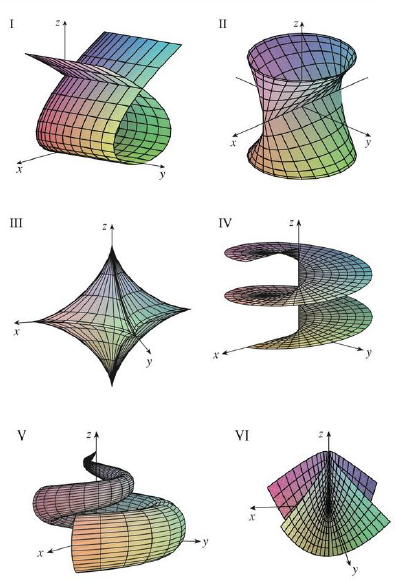Chapter 16.6, Problem 14E

Chapter
Section
Textbook Problem

Match the equations with the graphs labeled I–VI and give reasons for your answers. Determine which families of grid curves have u constant and which have v constant.14. r(u, v) = uv2 i + u2v j + (u2 − v2) kTo determine

To match: The equation r(u,v)=uv2i+u2vj+(u2v2)k with the graphs labeled I-VI in the textbook and to explain the reason for the combination.

Explanation

Given data:

The given vector equation as follows.

r(u,v)=uv2i+u2vj+(u2v2)k

The graphs are labeled in the textbook from I to VI.

Write the vector equation as follows.

r(u,v)=uv2i+u2vj+(u2v2)k

Write the parametric equations from the vector equation as follows.

x=uv2,y=u2v,z=u2v2 (1)

Determination of grid curves when u is constant:

Consider the parameter u as constant u0 .

Substitute u0 for u in equation (1),

x=u0v2,y=u02v,z=u02v2

From the expression y=u02v find the parameter v as follows.

v=(1u02)y

Substitute (1u02)y for v in the expression x=u0v2 ,

x=u0[(1u02)y]2=(1u03)y2

As u0 is considered as constant, consider (1u03) as a constant k .

Therefore, the expression x=(1u03)y2 is written as follows.

x=ky2 (2)

The expression represents the equation of parabola with the axis as x-axis.

Find the v2 from the expression x=u0v2 .

v2=(1u0)x

Substitute (1u0)x for v2 in the expression z=u02v2 ,

z=u02(1u0)x (3)

From equation (2) and (3), each grid curve corresponds to u=u0 (constant), that lies in the plane z=u02(1u0)x and the projection of each grid curve onto the xy-plane is a parabola with the axis as x-axis

Still sussing out bartleby?

Check out a sample textbook solution.

See a sample solution

The Solution to Your Study Problems

Bartleby provides explanations to thousands of textbook problems written by our experts, many with advanced degrees!

Get Started